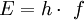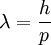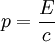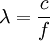# Is light a wave or a particle

Under Wave-particle dualism one understands a classical explanation of quantum mechanics, which says that objects from the quantum world can in some cases only be described as waves, in others as particles. With the interpretation of the statistical probabilities in the context of the Copenhagen interpretation, the term got a slightly different meaning: Each Radiation has both the character of waves and particles, but one or the other appears depending on the experiment being carried out.

### The wave nature of light

Over the years, different answers have been given to the question of whether light consists of particles or waves: Newton developed geometric optics in the 17th century on the assumption that light consists of particles. At the beginning of the 19th century, however, experiments by Young and Fresnel showed that light can be made to interfere, which of course was interpreted as a clear indication of its wave character. With the formulation of Maxwell's equations at the end of the 19th century and the resulting existence of electromagnetic waves that propagate at the speed of light, the wave nature of light was generally recognized.

### Einstein and the photons (light quanta)

In 1905 Albert Einstein again postulated to explain the photo effect that light should consist of "light quanta" (photons). In doing so, he was referring to Planck's work on the cavity radiator from 1900, in which the latter first assumed a quantization of the energy values ​​of the harmonic oscillator - for initially only mathematical considerations. The photon represents a single, so "discrete" portion of energy E. dar (quantization), so that the light can only absorb or release energy in integer multiples of this amount. The energy E. of a photon resulted from Planck's investigationsin which H is Planck's constant and f is the frequency of the photon.

This relationship also applies to mechanical waves, such as lattice vibrations in a solid.

### De Broglie and the wave character of particles

Louis de Broglie recognized in 1924 that particles with mass also have a wave character. He found out that a particle with the momentum p a wavelength λ ofowns. This equation also includes the equation for photons resulting from the special theory of relativity, for which applies,

with which for the wavelengthfollows. Here is c the speed of light. (More precisely: In the case of particles with mass, one would also have to consider the possibility of re-calibration.)

De Broglie's formula was experimentally confirmed in 1927 by diffraction of an electron beam on a nickel crystal by Davisson and Germer and finally by the electron double slit experiment by Claus Jönsson in 1961.

### Dissolution of the wave-particle dualism in quantum mechanics

In quantum mechanics, every particle is described by a wave function. The wave function of a particle is complex and therefore not a measurable variable. Only the square of the magnitude of the particle can be interpreted as the probability of its location (more precisely: as the volume density of the probability of its location) and can be determined in the experiment. The temporal development of the wave function of the particle and thus the change in its probability of being located is described by the Schrödinger equation.

### Quantum mechanics and statistical physics

On the microscopic level, the wave-particle dualism is the explanation for some physical phenomena. According to De Broglie, the wavelength of a particle depends on its speed and thus also on its temperature. At low temperatures, the De Broglie wavelengths of atoms can become larger than the atomic diameter and overlap, which partly explains the effects of the superfluidity of helium-3 and helium-4.

The wave character of the particles does not show up in macroscopic objects, which has two principal causes:

• Even when moving slowly, macroscopic objects, because of their large mass, have a wavelength that is considerably smaller than the dimensions of the object. In this case, the entire object can no longer be treated as a quantum mechanical object, but its components have to be described separately.
• In macroscopic objects there are permanent thermodynamically irreversible processes and photons (thermal radiation) are exchanged with the environment. Both lead to the decoherence of the system, which means that a state that may initially be capable of interference changes very quickly into one that is not capable of interference, which then behaves like a classic particle, i.e. not like a wave.